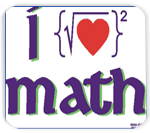## Math VocabularyIt's important to know the right math vocabulary when speaking about mathematics in class. This page provides math vocabulary for basic calculations.

Basic Math Vocabulary

+ - plus

Example:

2 + 2
Two plus two

- - minus

Example:

6 - 4
Six minus four

x OR * - times

Example:

5 x 3 OR 5 * 3
Five times three

= - equals

Example:

2 + 2 = 4
Two plus two equals four.

< - is less than

Example:

7 < 10
Seven is less than ten.

> - is greater than

Example:

12 > 8
Twelve is greater than eight.

≤ - is less than or equal to

Example:

4 + 1 ≤ 6
Four plus one is less than or equal to six.

- is more than or equal to

Example:

5 + 7 ≥ 10
Five plus seven is equal to or greater than ten.

- is not equal to

Example:

12 ≠ 15
Twelve is not equal to fifteen.

/ OR ÷ - divided by

Example:

4 / 2 OR 4 ÷ 2
four divided by two

1/2 - one half

Example:

1 1/2
One and one half

1/3 - one third

Example:

3 1/3
Three and one third

1/4 - one quarter

Example:

2 1/4
Two and one quarter

5/9, 2/3, 5/6 - five ninths, two thirds, five sixths

Example:

4 2/3
Four and two thirds

% - percent

Example:

98%
Ninety eight percent